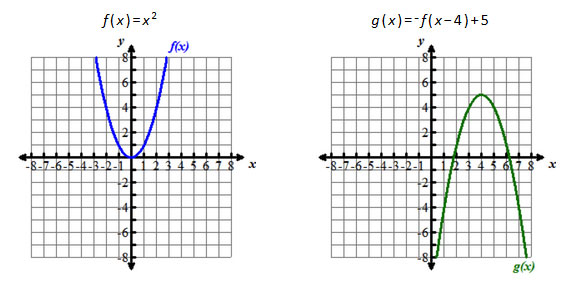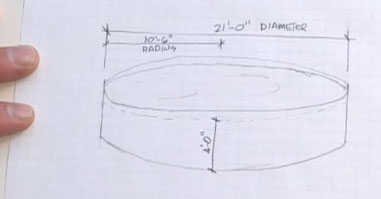## Middle School Math

Browse our collection of middle school math lessons, which include engaging interactive games as well as short, standard-focused lessons.

#### Algebra I LessonsExplore our collection of self-paced 20-minute lessons on Algebra I standards.

#### Middle School MathThis collection of interactive self-paced math lessons aligns to the Common Core State Standards in Mathematics and is part of a larger "Math at the Core" collection on PBS Learning Media.

> Go to Middle School Math

### Lure of the Labyrinth

In this game using pre-algebra skills, middle school students must work through puzzles to find their lost pet and save the world from monsters.

### Math by Design

Students apply geometry and measurement concepts as they take on the role of junior architects to create a town park and an environmental center.

### Sense and Dollars

Teach students about personal economics using this fun and interactive website.

### Villainy, Inc

In James Bond fashion, middle school students use their problem-solving acumen to foil the plans of the evil Dr. Wick in animated missions that require critical thinking and math calculations.

### KidVid: Fractions and Scale

In this interactive lesson, students consider how fractional measurements relate to scale models, learn about alternate ways of expressing scale ratios, and demonstrate understanding of fractions and scale.

### Popcorn Picker: Volumes of Solid Shapes

In this interactive lesson, students make predictions and experiment with the volume of two cylinders made from the same size paper.

### Square-Wheeled Tricycle: Radius and Circumference

In this interactive lesson, students work with the radius of various circles to find the circumference and area. They are challenged to find the distance a square wheel travels around a track.

### Cooking with Bill Nye: Dependent and Independent Variables

In this interactive lesson, students focus on dependent and independent variables to make a predictions about boiling water with the lid on or off the pot.

### Linear and Exponential Growth

In this interactive lesson, students practice solving exponential problems, comparing graphs of linear and making their own graphs of linear and exponential equations.

### Pairs of Linear Equations

In this interactive lesson, students focus on solving systems of equations by visualizing the problem or writing expressions with the Visualize It! tool.

### Comparing Powers of 10

In this interactive lesson, students use powers of 10 to investigate scientific notation.

### Multiplying and Dividing with Negatives

In this interactive lesson, students practice finding the products and quotients of positive numbers, negative numbers, and fractions and then finding the solutions on a number line.

### Real-World Ratio and Rate Reasoning: How to Power the Skate Park

In this interactive lesson, students view and analyze a series of video clips from Cyberchase and complete an activity using area and ratio.

### Using Sampling and Statistics to Get Rid of the Trash in Perfectamundo

In this interactive lesson, students use sampling, multiplication, and fractions to determine the amount of trash for removal. Students also complete a sampling and data analysis activity.

### Analyze the Data: Big Bay

In this interactive lesson, students will represent the data that they collect in a double bar graph in order to make comparisons and analyze the factors leading to the problem.

### Think Math- Wild Fractions

This collection includes standards-based interactives and videos that feature visual models for fractions, multiplication, and division.

### Think Math- Logical Leaps

This collection includes standards-based interactives about operations, number lines and graphing inequalities.

### Think Math- Maritime Mysteries

This collection includes standards-based interactives involving Cartesian graphs and the Pythagorean Theorem.